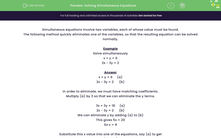# Solving Simultaneous Equations

In this worksheet, students solve simple simultaneous equations by eliminating one variable.Key stage:  KS 3

Curriculum topic:   Algebra

Curriculum subtopic:   Understand Expressions, Equations, Inequalities, Terms and Factors

Difficulty level:#### Worksheet Overview

Simultaneous equations involve two variables, each of whose value must be found.

The following method quickly eliminates one of the variables, so that the resulting equation can be solved normally.

Example

Solve simultaneously

x + y = 6

2x - 3y = 2

x + y = 6     (a)

2x - 3y = 2      (b)

in order to eliminate, we must have matching coefficients.

Multiply (a) by 3 so that we can eliminate the y terms.

3x + 3y = 18     (a)

2x - 3y = 2      (b)

We can eliminate y by adding (a) to (b)

This gives 5x = 20

So x = 4

Substitute this x value into one of the equations, say (a) to get

4 + y = 6

y = 2

Check by putting this into the other equation (b) to get 2 x 4 - 3 x 2 = 8 - 6 = 2

Solution is x = 4, y = 2

### What is EdPlace?

We're your National Curriculum aligned online education content provider helping each child succeed in English, maths and science from year 1 to GCSE. With an EdPlace account you’ll be able to track and measure progress, helping each child achieve their best. We build confidence and attainment by personalising each child’s learning at a level that suits them.

Get started